Imagine we are using power query to clean data and need to replace values as part of the transformation process.

For each value which we want to replace, we have to create a Replace Values step in the query. If we have tens or even hundreds of values to replace, then it can be quite tedious.

If we already have a list of values which we need to replace, then it would be easier if we could do it all in one step based on the list!

In this post we’re going to learn how to do a bulk find and replace in power query based on a list of values.

## The Setup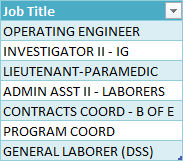In this example, we have a small table with one column called Job Title and the table has been named MyData. This is the data we’re going to transform with our find and replace function.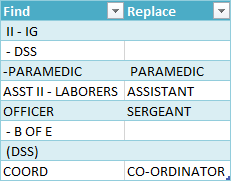We also have a table with two columns called Find and Replace and it’s been named MyFindReplace. This is a table of the pairs of items to find and replace.

The columns can actually be named anything, as we won’t be referring to them by name. The important thing is the find column is on the left and the replace column is on the right.

We then need to import these tables into power query. Go to the Data tab then press the From Table/Range command to import them into the power query editor.

## Using Replace Values In Power Query

When inside the power query editor, we can perform a Replace Values step from either the Transform tab or the Right Click menu.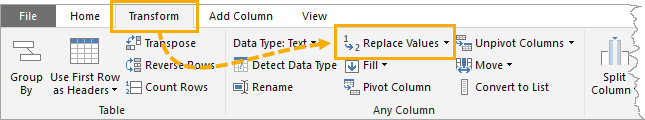Select the column which we want to replace values in and go to the Transform tab and press the Replace Values command.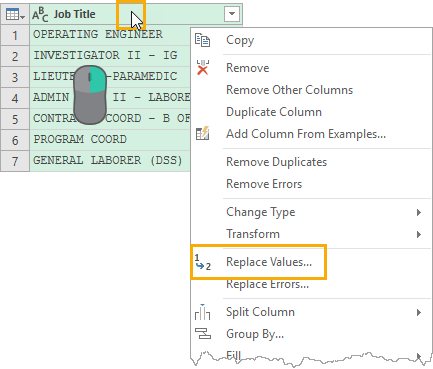We can also access this command by right clicking on the column heading then selecting Replace Values from the menu.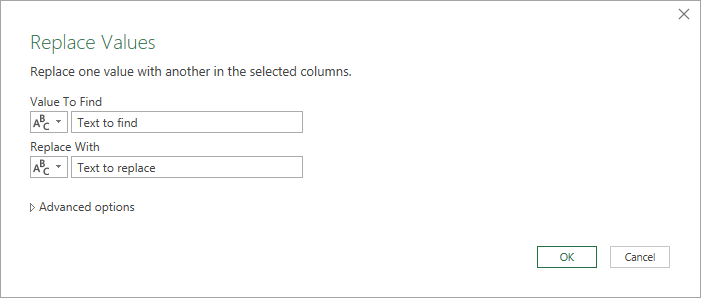Either of these options will open up the Replace Values menu and we can then enter the Value To Find and the value to Replace With.

This will then replace every instance of this in the entire column.

``= Table.ReplaceValue(#"Changed Type","Text to find","Text to replace",Replacer.ReplaceText,{"Job Title"})``

If we look at the M code that is generated from this, we can see it is using the Table.ReplaceValue power query function to perform the replacement.

We will use this function for our solution as well, but there is no easy way to use this function based on a list of values. We will need to create our own custom query function for this.

## M Code For The Query Function

``````let BulkReplace = (DataTable as table, FindReplaceTable as table, DataTableColumn as list) =>
let
//Convert the FindReplaceTable to a list using the Table.ToRows function
//so we can reference the list with an index number
FindReplaceList = Table.ToRows(FindReplaceTable),
//Count number of rows in the FindReplaceTable to determine
//how many iterations are needed
Counter = Table.RowCount(FindReplaceTable),
//Define a function to iterate over our list
//with the Table.ReplaceValue function
BulkReplaceValues = (DataTableTemp, n) =>
let
//Replace values using nth item in FindReplaceList
ReplaceTable = Table.ReplaceValue(
DataTableTemp,
//replace null with empty string in nth item
if FindReplaceList{n}{0} = null then "" else FindReplaceList{n}{0},
if FindReplaceList{n}{1} = null then "" else FindReplaceList{n}{1},
Replacer.ReplaceText,
DataTableColumn
)
in
//if we are not at the end of the FindReplaceList
//then iterate through Table.ReplaceValue again
if n = Counter - 1
then ReplaceTable
else @BulkReplaceValues(ReplaceTable, n + 1),
//Evaluate the sub-function at the first row
Output = BulkReplaceValues(DataTable, 0)
in
Output
in
BulkReplace``````

In a previous post about replicating Excel’s TRIM function in power query, we went through the steps to creating a query function. We will need to follow the same steps with the above M code.

This query function takes 3 arguments.

1. DataTable is the table that contains the columns which we want to find and replace values in.
2. FindReplaceTable is a two column table. The first column contains values to find and the second column contains values to replace them with. Each row in the table consists of one pair of find and replace values.
3. DataTableColumn is a list of the column names which we want to find and replace values in.

The function then converts our FindReplaceTable to a list of find and replace pairs and we iterate through them and apply a Table.ReplaceValue function to each pair.

## How To Use This Function

How we use this query function will be a bit different than our TRIM function example. In that case, we applied the function to each row in a column by adding a custom column.

To use this function, we need to apply it to the entire column.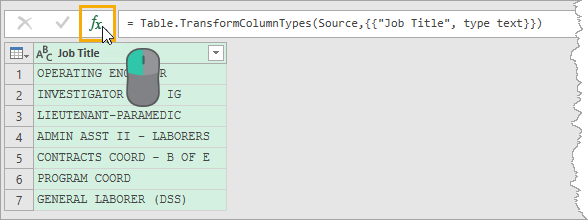We need to add a query step. From the query we want to use this function in, we can click on the small fx icon to the right of the formula bar.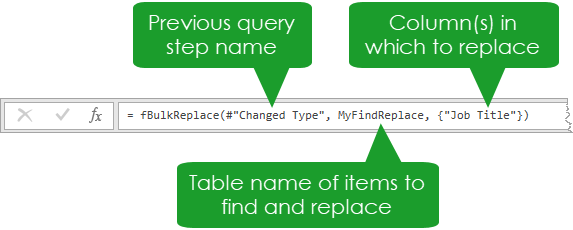``= fBulkReplace(#"Changed Type", MyFindReplace, {"Job Title"})``

We can then enter the above function.

The first argument will reference the previous step in the query. In our case this was a step named Changed Type.

The second argument will reference the name of the query which contains the table of find and replace values. In our case this was called MyFindReplace.

The third argument will reference the name of the column we want to replace values in. It needs to be in a list format using curly braces. In our case this was `{"Job Title"}`.

Note, that we could apply the find and replace to multiple columns using this argument. For example, placing `{"Job Title", "Job Description"}` in the third argument would apply the find and replace to both the Job Title and Job Description columns.

## Conclusions

With some ingenuity, we are able to create a function which can perform bulk find and replace steps based on a list of values.

The key is to create a recursive function based on the index of the list of find and replace values.

We can even use this solution to perform the find and replace on multiple columns simultaneously to save even more time.

Another great use of recursive query functions in power query!